# Projection Histogram of Binary Image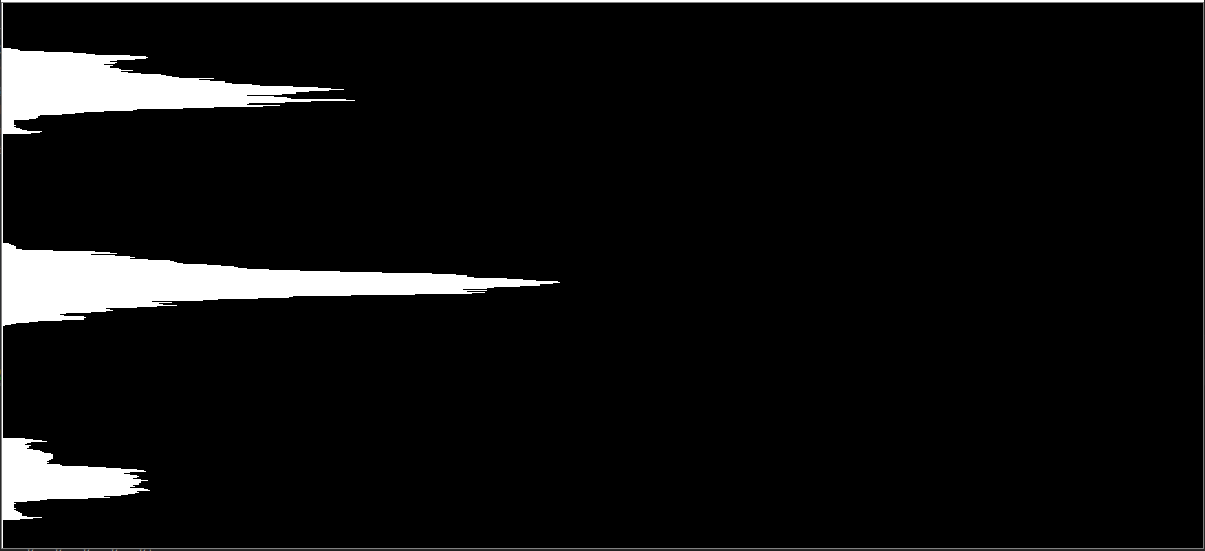figure 1 : Final Projection Histogram

For example, OCR projects needs to segment the lines, words and characters of the handwritten images to recognize the characters. For this segmenting part, they need to identify how is the projection is vary. According to that pattern they can split the main image and segment all the lines, words and characters.

First you have to choose a programming language to work with images. The most easiest and effective programming language for image processing is Python. Pycham is best IDE for coding with python. Ok lets move to the job.

# Requirements Need to Install

Python : After installing pycharm IDE, you have to install Python to your machine according to your Operating system(windows, Linux, mac).

PIP : Then you have to install PIP on python. Installing PIP is necessary because pip is a package management system used to install and manage software packages/libraries written in Python.

OpenCV : After installing python and pip, now you can install OpenCV library to you machine using the steps shown in here. Make sure that, to install OpenCV, you must preinstalled python and pip on your machine.

# Plotting Projection Histogram

Okay guys, lets move to our goal plotting histogram !!!

At first you have to create new python file on pycharm IDE using .py extension. Go to the “file” tab (which is showing upper left corner of the view and) and create a new file. Make sure give the file name with .py extension. I create my file as Image_Histgram.py , you can give any name here. After that you will get this view.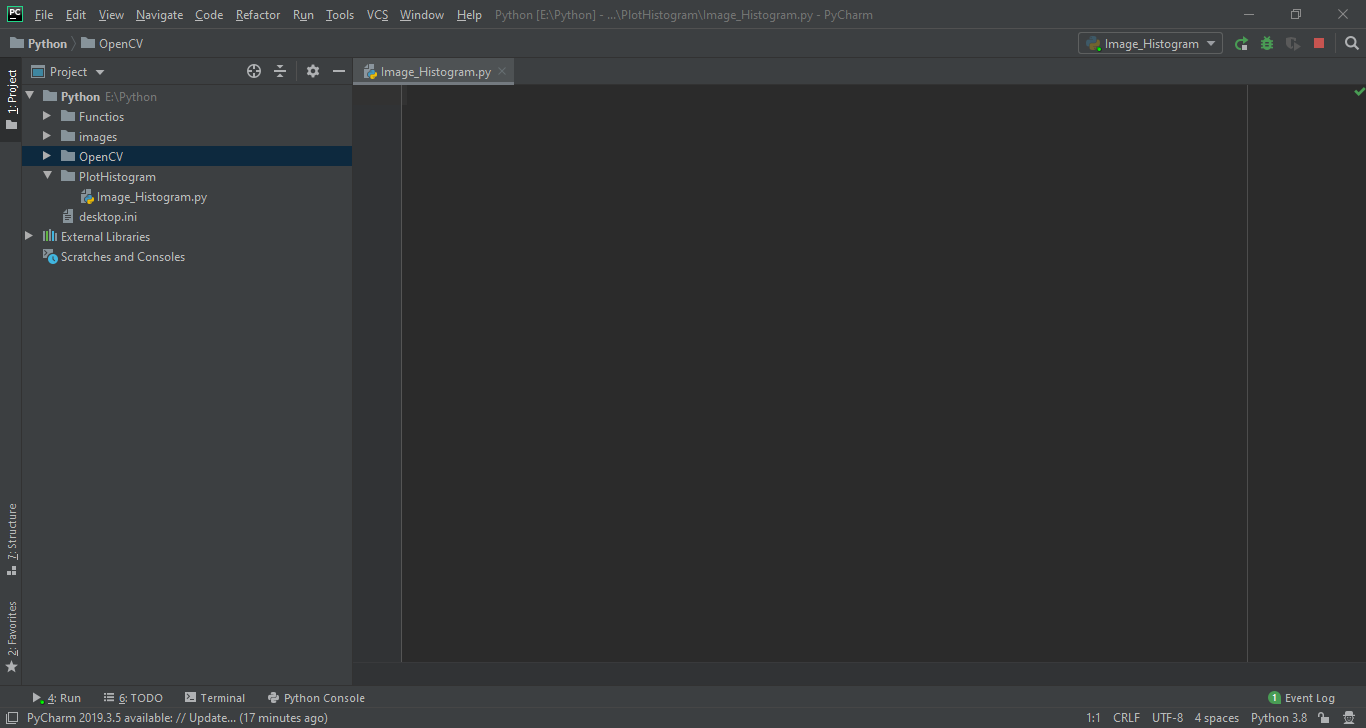figure 2: Pycharm script view

# Import libraries, Filtering and Thresholding

At first, it is necessary to import OpenCV and numpy packages to the script (Image_Histogram.py) using lines showing here.

`import cv2import numpy as np`

Next we can give our image which is need to get the histogram, as input to the system. To read the image, we use imread() function which is giving in opencv library. Here you should give the location of the image inside of imread function.

`inputImage = cv2.imread('E:/Final year project/Test Images/10.jpg')`

## Here is the image I have work with this chapter. It is a handwritten three sentences on a paper.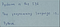figure 3: Input Image

Now you have load your image into the system. But to get projections of the image, we need to convert this image into grayscale image.

`grayImage= cv2.cvtColor(inputImage, cv2.COLOR_BGR2GRAY)`

Then we must add a filter to the image because most of the times natural images has some noises. To remove these noises we should add a filter. I have used Gaussian filter to remove noises.

`GaussianFilter= cv2.GaussianBlur(grayImage, (5,5), 0)`

Now we have to Threshold the image. By doing this, all the image color levels of the previous filtered image are categorized into two levels 0 and 255. Here I apply 127 as my threshold value, but according to the image type, you can change this.

`_, binarizedImage = cv2.threshold(GaussianFilter, 127, 255, cv2.THRESH_BINARY)`

The theory of applying the threshold value is, all the color levels below 127 are put into 0 th color level and all the color levels greater than 127 are put into 255 th color level.

After applying Thresholding technique, our image is shown like this.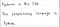figure 4: Image After applying Thresholding

# Calculate Projections

In the previous step we have threshold the image and named it as binarizedImage. At there we have put our image into only two color levels which is 0 and 255.

Here we put those 0th color value pixels (black pixels) into 1 and, 255th color value pixels (white pixels) into 0.

`binarizedImage[binarizedImage == 0] = 1binarizedImage[binarizedImage == 255] = 0`

Now our image consists black pixels (1) and white pixels(0) only.

After that using numpy package, we can easily calculate the projections of the image (vertical projection or horizontal projection). We use numpy.sum() function to get these projections. Inside of this function, we have to give our image name and axis.

If you wish to get horizontal projections of the image, you should give axis=1 to get it. axis=1 means this function go through the each row of the image and count how many black pixels were applying to each row separately. You also can see it when write a print function.

`horizontal_projection = np.sum(binarizedImage, axis=1);print(horizontal_projection);`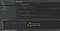figure 5: Horizontal Projections

This arrow shows you the horizontal projection of the image. To get vertical projections, you have to change axis=0. Then it go through every column and count black pixels sum or each column.

# Create Blank Image to plot Projection

To plot histogram, at first we need to create a blank image as same height and same width to our binarizedImage (Threshold Image). So we need to get height and width of the binarizedImage first.

we can use image.shape command to get height and width which is showing below.

`height, width = binarizedImage.shapeprint('width : ', width)print('height : ', height)`

Now we can create a blank image according to this height and width. We can use numpy.zeros() function to create blank image. We can simply create a blank image when giving relevant height and width.

`blankImage = np.zeros((height, width, 3), np.uint8)`

After creating a blank image, you will get this type of image. This is the image which we are used to plot projections of the image.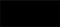figure 6: Blank Image

# Plot Projection Histogram

Finally, the last thing we have to do is go through each row or each column of the blank image and draw a line according to black pixel count which we have calculated previously. Then it gives us horizontal or vertical projections.

To plot horizontal projection . . .

`for row in range(height):    cv2.line(blankImage, (0,row), (int(horizontal_projection[row]*width/height),row), (255,255,255), 1)`

Here we implement a for loop to go through every row of the image. So we write a for loop for height of the image.

Then inside of the for loop, draw a line using cv2.line() function. It will draw lines for every separate row of the image according to black pixel count applied for each row.

Inside of the cv2.line() function, we should give five(5) parameters for it. Those parameters are, image name, start coordination for line, end coordination for line, color of the line, line thickness.

Finally the system gives us the projection profile of the image like this.(I have get horizontal projection here.)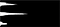figure 7 : Final Projection Histogram

See you in next chapter.

Thanks all !

Final year Undergraduate at Faculty of Information Technology, University of Moratuwa, Sri Lanka

## More from Sachith Sampath Priyashantha

Final year Undergraduate at Faculty of Information Technology, University of Moratuwa, Sri Lanka